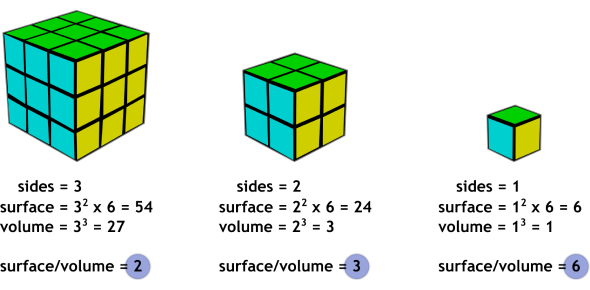# Surface Area, Volume, Area Quiz

21 Questions | Total Attempts: 4222Settings.

• 1.
I need to figure surface area for
• A.

How much tissue will fit in a tissue box

• B.

Wrapping a present

• C.

Table cloth

• D.

New necklace

• 2.
Surface Area is found by:
• A.

Adding the legnths of all the sides together

• B.

Adding the area of all the sides together

• C.

Multiplying the legnth and bases together

• D.

Multiplying the perimeter by two

• E.

None of the above

• 3.
Given a cylinder with a radius of 5 and a hight of seven, what is the surface area?
• A.

219.8 units squared

• B.

157 units squared

• C.

376.8 units squared

• D.

298.3 units squared

• E.

None of the above

• 4.
Find the surface area ofa pyramid with a square base legnth 10 and a slant height of 13
• A.

580 units squared

• B.

480 units squared

• C.

620 units squared

• D.

340 units squared

• E.

None of the above

• 5.
Find the total Surface area of a cone with radius 3, slant height of 5.
• A.

94.2 units squared

• B.

28.26 units squared

• C.

78.5 units squared

• D.

122.46 units squared

• E.

None of the above

• 6.
What is the volume of a rectangular prism that is 1 cm. by 2 cm. by 3 cm.?
• A.

6 cu. cm.

• B.

6 sq. cm.

• 7.
If you enlarge a rectangular prism with dimensions of 1 cm. by 2 cm. by 3 cm. by a scale factor of 3, what will its new dimensions be?
• A.

3 cm. by 6 cm. by 9 cm.

• B.

3 cm. by 5 cm. by 6 cm.

• 8.
How many square feet of sand can fit into a sandbox that is 8 feet by 8 feet and 1 foot high?
• A.

64 square feet

• B.

17 square feet

• 9.
How many square feet of cement will it take to fill a hole that is 6" by 3 feet by 6 feet?
• A.

108 sq. ft.

• B.

9 sq. ft.

• 10.
Which rectangular prism has a larger surface area? Prism A: Dimensions are 5 by 4 by 2 Prism B: Dimensions are 6 by 3 by 2
• A.

Prism A

• B.

Prism B

• C.

They have the same Surface Area

• 11.
Which rectangular prism has a larger volume? Prism A: Dimensions are 5 by 4 by 2 Prism B: Dimensions are 6 by 3 by 2
• A.

Prism A

• B.

Prism B

• C.

They have the same volume.

• 12.
What is the surface area of the rectangular prism?
• A.

358

• B.

420

• C.

179

• D.

210

• 13.
What is the Volume of the prism below:
• A.

358

• B.

420

• C.

179

• D.

210

• 14.
What is the surface area of the pyramid below?
• A.

216

• B.

648

• C.

225

• D.

324

• 15.
What is the volume of the pyramid below?
• A.

112

• B.

116.48

• C.

74.24

• D.

37.33

• 16.
What is the surface area of the cyliner?
• A.

96

• B.

1005.31

• C.

2412.74

• D.

804.25

• 17.
What is the volume of the cylinder below?
• A.

96

• B.

1005.31

• C.

2412.74

• D.

804.25

• 18.
What is the surface area of the cone below?
• A.

3446.4

• B.

703.72

• C.

742.42

• D.

1340.41

• 19.
What is the volume of the cone below?
• A.

3446.4

• B.

703.72

• C.

742.42

• D.

1340.21

• 20.
A rectangular prism has a volume of 120. Which dimensions can be the correct ones?
• A.

8*3*8

• B.

5*2*6

• C.

8*3*5

• 21.
To find the radius of a cylinder you should measure the diameter and divide by 2 ?
• A.

True

• B.

False

Related TopicsBack to top